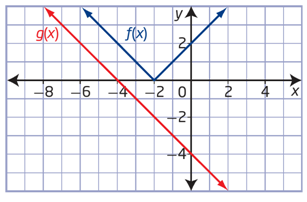# Composite functions

### Composite functions

#### Lessons

• Introduction
What is a "Composite Function"?
a)
Quick review on basic operations with functions.

b)
Composition of Functions: putting one function inside another function!

• 1.
Introduction to Composite Functions
If $f(x)= 4x-5$
$g(x)=8x^2+6$
Determine
a)
$(f\circ g)(x)$

b)
$(g\circ f)(x)$

c)
$(f\circ f)(x)$

d)
$(g\circ g)(x)$

e)
$(f\circ g)(2)$
evaluate in two different ways

• 2.
Evaluate a Composite Function Graphically
Use the graphs of $f(x)$ and $g(x)$ to evaluate the following:a)
$f(g(-4))$

b)
$f(g(0))$

c)
$g(f(-2))$

d)
$g(f(-3))$

• 3.
Determine the Composition of Three Functions
Use the functions $f(x)=3x,$
$g(x)=x-7$
and $h(x)=x^2$
to determine each of the following:
a)
$(f\circ g\circ h)(x)$

b)
$g(f(h(x)))$

c)
$f(h(g(x)))$

d)
$(h\circ g\circ f)(x)$

• 4.
Determine the Original Functions From a Composite Function
If $h(x)=(f\circ g)(x)$
determine $f(x)$
and $g(x)$
a)
$h(x)=(7x-5)^3-4(7x-5)+1$

b)
$h(x)=\sqrt{4x^3-9}$, give two possible sets of solutions

• 5.
Composite Functions with Restrictions
Consider $f(x)=\sqrt{x-13}$
and $g(x)=x^2+4$, for each of the function below:
a)
Determine:
i)
$(f\circ g)(x)$
ii)
$(g\circ f)(x)$

b)
State the domain and range of:
i)
$f(x)$
ii)
$g(x)$
iii) $(f\circ g)(x)$
iv)
$(g\circ f)(x)$

c)
Sketch the graph of:
i)
$f(x)$
ii)
$g(x)$
iii) $(f\circ g)(x)$
iv)
$(g\circ f)(x)$